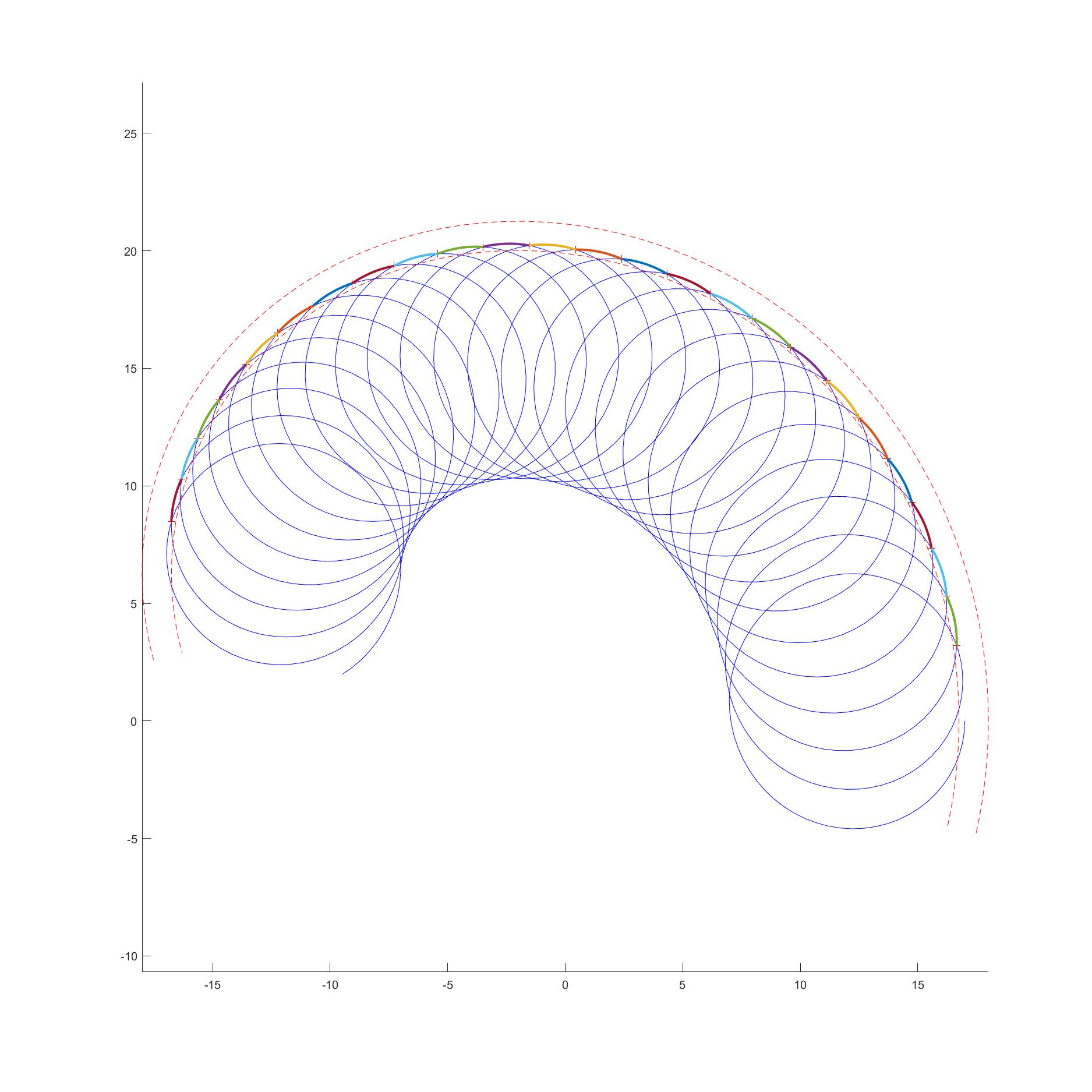# Trochoid – Outer Lobe Intersections

Finds the outermost intersection points for the following parametric function:

X(t) = R_cp * cos(Phi_dot * t) + R_t * cos(theta_dot * (-t))
Y(t) = R_cp * sin(Phi_dot * t) + R_t * sin(theta_dot * (-t)) + nu * t

% code runs efficienty by only checking points between an outer ring, defined by push_far_factor, and an inner ring, defined by push_close_factor. t_step_init_Factor adjust the spacing between points that are checked for intersections.

push_far_factor should be >1
push_close_factor should <1
t_step_init_Factor is generally a value between 5 and 30, but should be set as low as possible for optimal efficiency

push_far_factor, push_close_factor, and t_step_init_Factor can be defined iteratively. After observing the solution plot, there should be rings (red dashed line) located just outside of the outer lobe and just inside the outer lobe. If the plot looks choppy (i.e. low resolution) or points have been missed, the t_step_init_Factor should be increased.